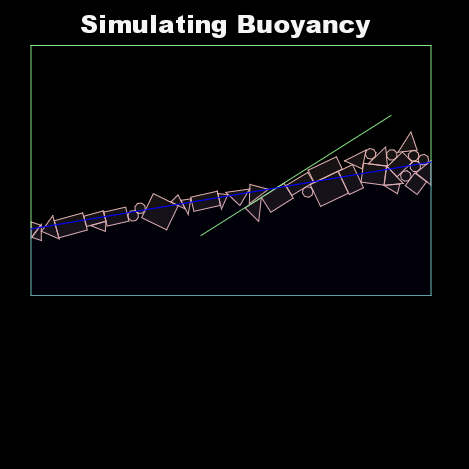Get access to over 100 FireMonkey cross platform samples for Android, IOS, OSX, Windows, and Linux!

# Simulate Liquid Buoyancy With Box2d In Delphi XE6 Firemonkey On Android And IOSOne of the sample projects included with Box2d Firemonkey for Delphi XE5 & XE6 on Android and IOS is a Buoyancy Controller project. The code also works cross platform on Windows and OSX. The Buyancy Controller demo shows you how to give setup an object to simulate water and how to assign buoyance to your objects so that they will float in the simulated water. Box2d Firemonkey is a full featured physics engine already so adding this functionality to your project is pretty simple. It might be interesting to modify this project so that the water in the demo is based on the mobile device orientation. Here is some sample code for setting up the buoyancy values on a Box2d body:
```bodyDef := Tb2BodyDef.Create; bodyDef.bodyType := b2_dynamicBody; fd := Tb2FixtureDef.Create; fd.shape := m_polygons[idx]; fd.density := density; // Override the default friction. fd.friction := 0.3; fd.restitution := 0.1; SetValue(bodyDef.position, RandomFloat(-18, 18), RandomFloat(4, 13)); bodyDef.angle := RandomFloat * Pi; body := m_world.CreateBody(bodyDef); body.CreateFixture(fd, True, False);```
And here is the sample code from the Step function which shows how the water is handled and drawn:
```procedure TBuoyancyController.Step(var settings: TSettings; timeStep: PhysicsFloat); const waterlevel: RGBA = (0.0, 0.0, 1.0, 1.0); waterarea: RGBA = (0.0, 0.0, 1.0, 0.3); var theta: PhysicsFloat; vertices: array[0..3] of TVector2; begin time := time + timeStep; if wave then begin theta := 10 * Sin(time / 2) / 180 * Pi; // 10 is the max angle of wave SetValue(bc.normal, -Sin(theta), Cos(theta)); end else theta := 0;```

``` inherited; vertices := MakeVector(-20, -10); vertices := MakeVector(-20, -20 * Tan(theta)); vertices := MakeVector(20, 20 * Tan(theta)); vertices := MakeVector(20, -10); m_world.Draw.DrawSolidPolygon((Pb2PolyVertices(@vertices))^, 4, waterarea); m_world.Draw.DrawSegment(vertices, vertices, waterlevel); ```